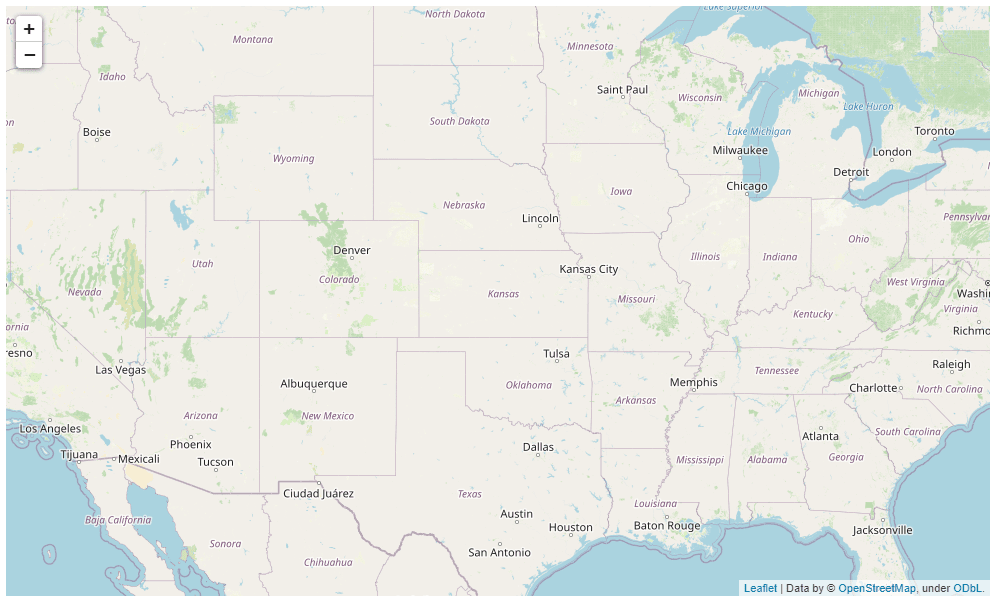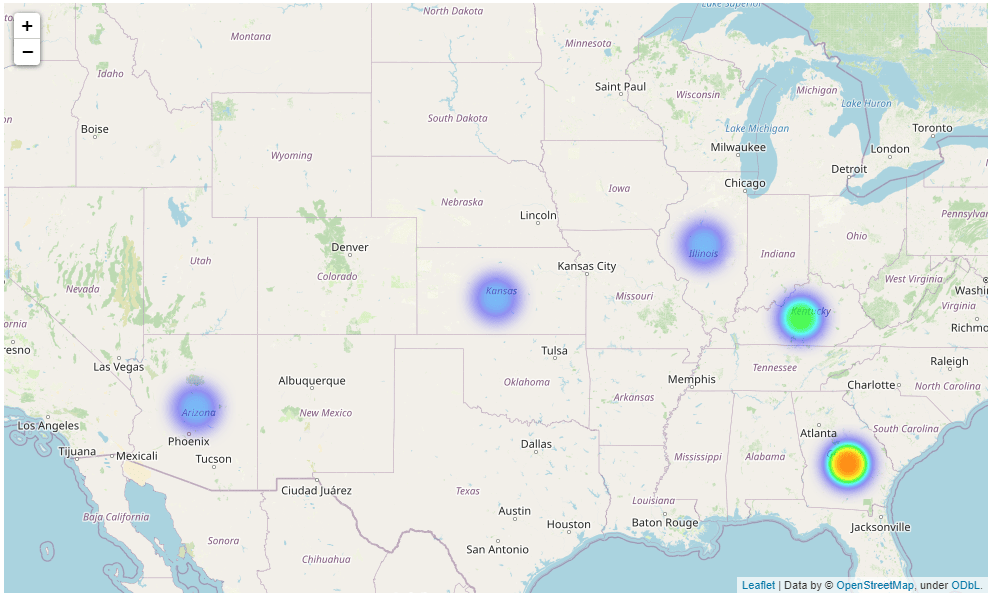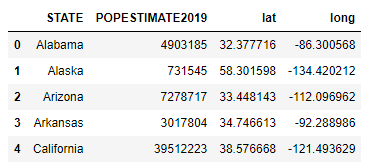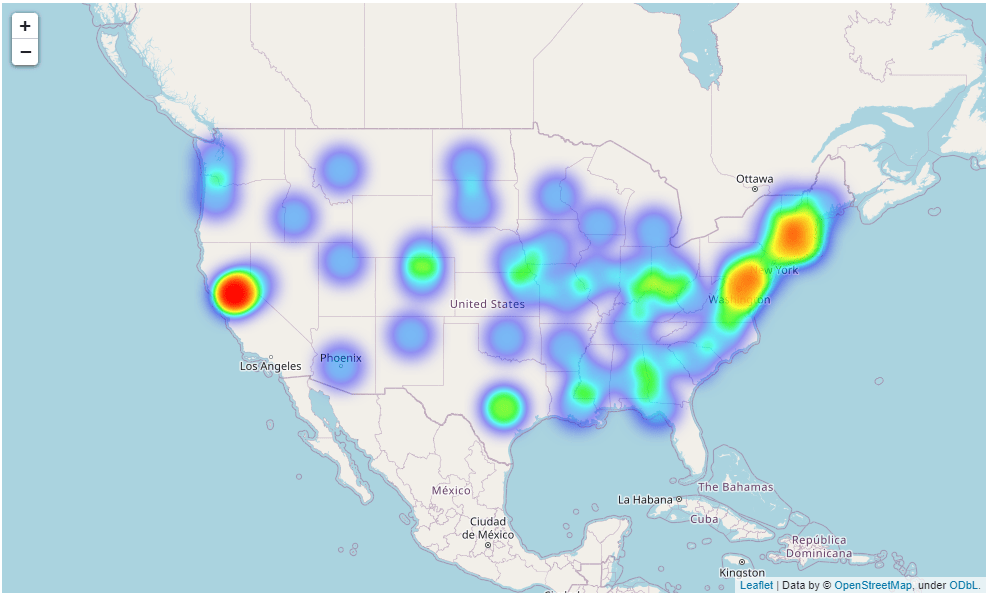This tutorial will show you how to plot geographical heatmaps with the Python Folium module. We’ll explain how to plot a heatmap using latitude/latitude coordinates of a location or using location names. Finally, we’ll walk through a real-life example of plotting geographical heatmaps displaying the population of different US states.

## Installing Folium

You can use the following pip command to install the Python folium module.

``pip install folium``

## Plotting a Simple Map with Folium

The `Map` class from the folium module plots a blank geographical map. To center a map around a specific geographical location, you need to pass the latitude and longitude values of the location in a list to the `location` attribute of the `Map` class constructor.

You can also set the zoom level by passing an integer value to the `zoom_start` attribute. The following script plots a simple folium map centered around the US state of Kansas.

``````import folium

map_obj = folium.Map(location = [38.27312, -98.5821872], zoom_start = 5)

map_obj``````

OutputYou can save a folium map by calling the `save()` function on the map object. Here’s an example:

``````import folium

map_obj = folium.Map(location = [38.27312, -98.5821872], zoom_start = 5)

map_obj.save(r"C:\Datasets\folium_map.html")``````

Notice how we’re able to save it as an html file that allows us to pan and zoom our image after we save it.

I put together a Python Developer Kit with over 100 pre-built Python scripts covering data structures, Pandas, NumPy, Seaborn, machine learning, file processing, web scraping and a whole lot more - and I want you to have it for free. Enter your email address below and I'll send a copy your way.

Yes, I'll take a free Python Developer Kit

## Plotting a Heatmap using Latitude/Longitude Values

The folium `HeatMap` class plots a geographical heatmap in Python. To do this, you first need to create an object of the `Map` class, like we did in our previous script.

Next, you need to create a list of lists containing latitude and longitude values of the locations that you want to plot on the heatmap.

The latitude and longitude list is passed to the `HeatMap` class.

Finally, you need to add the `Map` class object to the `Heatmap` class using the `add()` method, as shown in the script below.

The following script plots a heatmap with markers on five US states: Kansas, Arizona, Kentucky, Georgia, and Illinois.

``````import folium

from folium.plugins import HeatMap

map_obj = folium.Map(location = [38.27312, -98.5821872], zoom_start = 5)

lats_longs = [
[38.27312, -98.5821872], # Kansas
[34.395342, -111.763275], # Arizona
[37.5726028, -85.1551411], # Kentucky
[32.3293809, -83.1137366], # Georgia
[40.0796606, -89.4337288], # Illiniois
]

map_obj``````

Output## Adding Weights to a Heatmaps

As you would imagine, you can pass weight values for locations on your heatmap - that’s the point of a heatmap. The spots for locations with greater weight values will have a higher color intensity compared to those locations with lower weight values.

A weight value for a location is passed as the third item in the list containing latitude and longitude values. Here’s an example:

``````import folium

from folium.plugins import HeatMap

map_obj = folium.Map(location = [38.27312, -98.5821872], zoom_start = 5)

lats_longs = [
[38.27312, -98.5821872, 0.5], # Kansas
[34.395342, -111.763275,0.2], # Arizona
[37.5726028, -85.1551411, 0.7], # Kentucky
[32.3293809, -83.1137366,0.9], # Georgia
[40.0796606, -89.4337288,0.1], # Illinois
]

map_obj``````

You can see from the output that some locations now appear brighter, or more intense, than others.I put together a Python Developer Kit with over 100 pre-built Python scripts covering data structures, Pandas, NumPy, Seaborn, machine learning, file processing, web scraping and a whole lot more - and I want you to have it for free. Enter your email address below and I'll send a copy your way.

Yes, I'll take a free Python Developer Kit

## Plotting a Heatmap using Location Names

Though the `HeatMap` class constructor accepts a list of lists containing latitude and longitude values, you can also plot a folium `HeatMap` using location names.

To do so, you can use the `Nominatim` class from the Geopy module to get latitude and longitude values of a particular location. If you don’t have the geopy module installed, you’ll need to install it with a command like the following:

``pip install geopy``

The latitude and longitude coordinates can be then passed to your `HeatMap` class.

The following example shows how you can get latitude and longitude values using a location name, with the help of the `Nominatim` class. The script finds the latitude and longitude coordinates for the US state of Kansas.

``````from geopy.geocoders import Nominatim

geolocator = Nominatim(user_agent="bytescout", timeout=None)

location = "Kansas"

loc_lat_long = geolocator.geocode(query = location)

print(loc_lat_long.latitude, loc_lat_long.longitude)``````

Output

``38.27312 -98.5821872``

Now that we have a tool for grabbing geographical coordinates, let’s see an example of how location names can be used to plot heatmaps. The script below defines a function named `getloc_lats_longs()` that accepts a list of location names as parameter values. It then returns a list of lists containing latitude and longitude coordinates for the specified locations.

``````from geopy.geocoders import Nominatim

geolocator = Nominatim(user_agent="bytescout", timeout=None)

def getloc_lats_longs(locations):

loc_lats_longs = []

for loc in locations:

loc_lat_long = geolocator.geocode(query = loc)

loc_lats_longs.append([loc_lat_long.latitude, loc_lat_long.longitude])

return loc_lats_longs``````

This next script passes a list containing names of five US states to the `getloc_lats_longs()` function, which returns latitude and longitude coordinates for those states.

The list returned by the `getloc_lats_longs()` function is passed to the `HeatMap` class constructor, which displays a heatmap.

``````import folium

from folium.plugins import HeatMap

states = [ "Kansas", "Arizona", "Kentucky", "Georgia", "Illinois"]

map_obj = folium.Map(location = [38.27312, -98.5821872], zoom_start = 5)

lats_longs = getloc_lats_longs(states)

map_obj``````

Output## Plotting a Heatmap For US States Population

Alright, now that we’ve walked through the basics, let’s use these new skills to solve a real-life example where we need to plot populations for US states.

We’re going to make a geographical heatmap using 2019 estimates of state population in the US. The dataset is available at this Kaggle link but we mirrored it here for your convenience.

The following script imports the dataset into Pandas using the read_csv function and displays the first few lines.

``````import pandas as pd

OutputThe dataset contains four columns, as you can see from the above output. For latitude and longitude, we’ll be pulling values from the lat and long columns, which represent the location of the state capitals for each state. The POPESTIMATE2019 column contains population values for the 50 states (and Washington DC). These values will be used as weights for our `HeatMap` class constructor.

The following script creates a list of lists containing the latitude, longitude and weights (population values) for all the states in the dataset. The first five values from the list of lists are displayed.

``````lats_longs_weight = list(map(list, zip(population_data["lat"],
population_data["long"],
population_data["POPESTIMATE2019"]
)
)
)
lats_longs_weight[:5]``````
``````[[32.377716, -86.300568, 4903185],
[58.301598, -134.420212, 731545],
[33.448143, -112.096962, 7278717],
[34.746613, -92.288986, 3017804],
[38.576668, -121.493629, 39512223]]``````

Finally, the list of lists containing latitude, longitude and weight values is used to plot heatmaps, as shown in the script below:

``````import folium

from folium.plugins import HeatMap

map_obj = folium.Map(location = [38.27312, -98.5821872], zoom_start = 4)

map_obj``````

The output below shows the 2019 population estimates as a heatmap for all the US states. That’s pretty neat, isn’t it?

Output